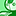# QlikView App Dev

Discussion Board for collaboration related to QlikView App Development.

Announcements
Join us at the Cloud Data and Analytics Tour! REGISTER TODAY
cancel
Showing results for
Search instead for
Did you mean:Partner

## help with min/max and avg on chart

hi all, i have a chart in the attached qvw which has an expression giving me actuals.

I need to add expressions to show the min/ max and avg but i am not getting much luck in getting it working.

Can anyone help please?

1 Solution

Accepted SolutionsMVP

=Min(Aggr(COUNT(Type),Hour))

=Max(Aggr(COUNT(Type),Hour))

=Avg(Aggr(COUNT(Type),Hour))

=Min(Aggr(Count (Type)/Count(DISTINCT Date),Hour))

=Max(Aggr(Count (Type)/Count(DISTINCT Date),Hour))

=Avg(Aggr(Count (Type)/Count(DISTINCT Date),Hour))

If you want to show for TOTAL.. use as below

=Min(TOTAL......)

=Max(TOTAL.....)

=Avg(TOTAL......)

3 RepliesMVP

=Min(Aggr(COUNT(Type),Hour))

=Max(Aggr(COUNT(Type),Hour))

=Avg(Aggr(COUNT(Type),Hour))

=Min(Aggr(Count (Type)/Count(DISTINCT Date),Hour))

=Max(Aggr(Count (Type)/Count(DISTINCT Date),Hour))

=Avg(Aggr(Count (Type)/Count(DISTINCT Date),Hour))

If you want to show for TOTAL.. use as below

=Min(TOTAL......)

=Max(TOTAL.....)

=Avg(TOTAL......)Master II

Hi,

Add these as Expression

=Min(Aggr(COUNT(Type),Hour))

=Max(Aggr(COUNT(Type),Hour))

=Avg(Aggr(COUNT(Type),Hour))

And in dimension put:

User

That will give you 3 lin charts for all users.

Regards

KC

Best Regards,
KCMaster II

Sorry, use this:

=Min(Aggr(COUNT(Type),User))

=Max(Aggr(COUNT(Type),User))

=Avg(Aggr(COUNT(Type),User))

And in dimension use:

User

Best Regards,
KC